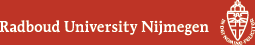## Geometry Seminar - Abstracts

### Talk

Thursday 17 January 2019, 16:00-17:00 in HG00.310
Otto Overkamp (Hannover)
Kulikov models of Kummer surfaces

### Abstract

Let $$K$$ be a complete discrete valuation field with algebraically closed residue field $$k$$; we assume that the characteristic of $$k$$ is different from $$2$$. Let $$X$$ be a K3 surface over $$K$$, i.e. a smooth, projective, and geometrically integral algebraic surface over $$K$$ with trivial canonical sheaf and trivial $$H^1(X, \mathcal O_X)$$. In general, it is an open question whether we can find a finite extension of $$K$$ such that there exists a semistable model of $$X$$ over the ring of integers of that finite extension, even if we allow the model to be an algebraic space rather than a scheme. I shall explain how the question can be answered affirmatively if $$X$$ is the Kummer surface associated with some Abelian surface over $$K$$. In fact, we can even show that the models we construct are schemes, and that their relative canonical sheaf vanishes (i.e., the models we construct are so-called Kulikov models). Time permitting, I shall say a few words about the general theory of Kulikov models.

(Back to geometry seminar schedule)